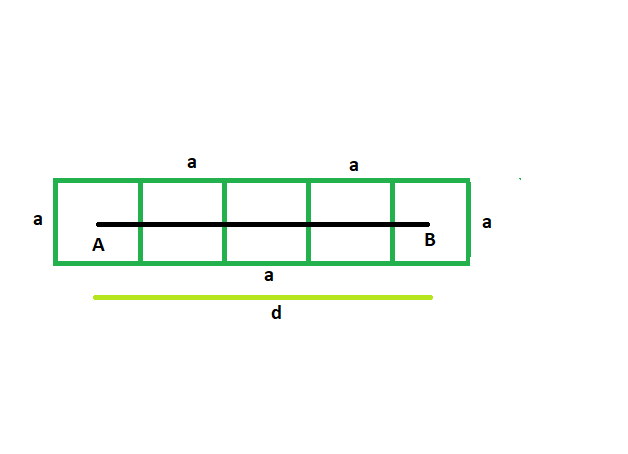# Find the side of the squares which are lined in a row, and distance between the centers of first and last square is given

• Last Updated : 31 May, 2022

Given here are n squares which touch each other externally, and are lined up in a row. The distance between the centers of the first and last square is given. The squares have equal side length. The task is to find the side of each square.
Examples:

```Input: d = 42, n = 4
Output: The side of each square is 14

Input: d = 36, n = 5
Output: The side of each square is 9```Approach:
Suppose there are n squares each having side of length a
Let, the distance between the first and last squares = d
From the figure, it is clear,
a/2 + a/2 + (n-2)*a = d
a + na – 2a = d
na – a = d
so, a = d/(n-1)## C++

 `// C++ program to find side of the squares` `// which are lined in a row and distance between the` `// centers of first and last squares is given`   `#include ` `using` `namespace` `std;`   `void` `radius(``int` `n, ``int` `d)` `{` `    ``cout << ``"The side of each square is "` `         ``<< d / (n - 1) << endl;` `}`   `// Driver code` `int` `main()` `{` `    ``int` `d = 42, n = 4;` `    ``radius(n, d);` `    ``return` `0;` `}`

## Java

 `// Java program to find side of the squares` `// which are lined in a row and distance between the` `// centers of first and last squares is given` `import` `java.io.*;`   `class` `GFG` `{`   `static` `void` `radius(``int` `n, ``int` `d)` `{` `    ``System.out.print( ``"The side of each square is "` `        ``+ d / (n - ``1``));` `}`   `// Driver code` `public` `static` `void` `main (String[] args) ` `{` `    ``int` `d = ``42``, n = ``4``;` `    ``radius(n, d);` `}` `}`   `// This code is contributed by vt_m.`

## Python3

 `    `  `# Python program to find side of the squares` `# which are lined in a row and distance between the` `# centers of first and last squares is given`   `def` `radius(n, d):`   `    ``print``(``"The side of each square is "``,` `          ``d ``/` `(n ``-` `1``));`     `d ``=` `42``; n ``=` `4``;` `radius(n, d);`       `# This code contributed by PrinciRaj1992`

## C#

 `// C# program to find side of the squares` `// which are lined in a row and distance between the` `// centers of first and last squares is given` `using` `System;`   `class` `GFG` `{`   `static` `void` `radius(``int` `n, ``int` `d)` `{` `    ``Console.Write( ``"The side of each square is "` `        ``+ d / (n - 1));` `}`   `// Driver code` `public` `static` `void` `Main () ` `{` `    ``int` `d = 42, n = 4;` `    ``radius(n, d);` `}` `}`   `// This code is contributed by anuj_67..`

## Javascript

 ``

Output:

`The side of each square is 14`

Time Complexity: O(1)

Auxiliary Space: O(1)

My Personal Notes arrow_drop_up
Recommended Articles
Page :